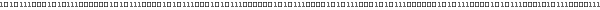# Tutorial 04 : Draw simple rotating shapes - Render• ## Tutorial

Now, we can begin the OpenGl tutorials. All the tutorials will runs eiher with Gl4java, Jogl and Jogl JSR231.

This first tutorials will learn you how to draw simple shapes like triangles, quads.
By association of simple 2D shapes, we can creates 3D shapes like a pyramid (composed of 4 triangles and 1 quad) and a cube (composed of 6 quads).
We will also view how to add colors and see the differents render : point, linear and fill.

• ### Introduction

To draw a simple shape like a triangle, we need the coordinates his 3 vertices.
These vertices are marked in white in the following picture :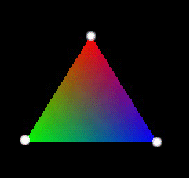In a 3D world, coordinates of a vertex are represented by 3 values (x, y, z) in the axis system :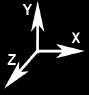x is the position in the X axis, y is the position on the Y axis ...
The origin (the center) of the axis system is the point (0, 0, 0). This point is in the center of the scene (this is done when reseting transformation matrix with glLoadIdentity). We can apply transformations such as translation, rotation and scaling to the axis system.

By combining tringles, we can creates the pyramid shown on the screen shot of the tutorial.
For the cube, we will use a combination of quads.

• ### The Pyramid

Here is the pyramid represented with its axis system, vertices are marked with white points :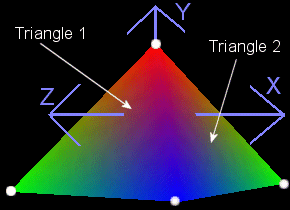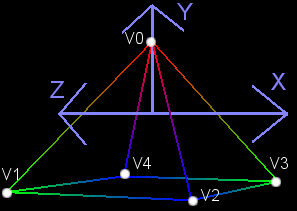Pyramid rendered with GL_FILL Pyramid rendered with GL_LINE

To draw this pyramid, we will draw each of its 4 triangles successively.
The pyramid have 5 differents vertices that will be called V0, V1, V2 ...

• #### The Drawing

The first triangle is composed of the vertex V0, V1 and V2, which have the coordinates :
V0 = ( 0,  1, 0)
V1 = (-1, -1, 1)
V2 = ( 0, -1, 1)

Each vertices have a color. The color used by OpenGL is in RGB mode. The color is also composed of 3 components : (r, g, b).
Each component defines the percentage of red(r), green(g) and blue (b). The value of each component must be include in the interval [0.0f, 1.0f]
V0 is affected by the color red   = (1.f, 0.f, 0.f)    //r=1 means full red
V1 is affected by the color green = (0.f, 1.f, 0.f)    //g=1 means full green
V2 is affected by the color blue  = (0.f, 0.f, 1.f)    //b=1 means full blue

Here is some examples forOther colors :
whith = (1.f, 1.f, 1.f)      //full of red, green and blue make white
black = (0.f, 0.f, 0.f)      //no color

To draw a vertex (x, y, z), we use the OpenGl method :
gl.glVertex3f(x, y, z)
The color affected to this vertex is the current color defined. To affect a color on a vertex, we simply set the current color before calling glVertex. This is done with :
gl.glColor3f(r, g, b)      //Default : white

The calls glVertex must be placed into a bloc glBegin / glEnd. With this block, we tells OpenGl that want to draw primitive shape.
The kind of shape drawn is specified with the parameter of glBegin. The following picture, taken from The Red Book, shows all primitive shape with their corresponding parameter :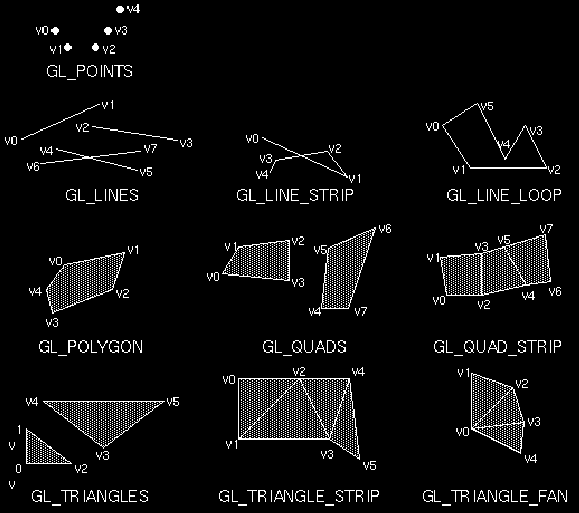Picture Copyrigth @ Addison-Wesley Publishing Company

For drawing a pyramid, we can either use :

• GL_TRIANGLE     Draw sucessively 4 independant triangles, this will result on drawing 12 vertices.

• GL_TRIANGLE_FAN Take the advantage of common vertices of the pyramid, this will result on drawing 6 vertices.

Here is two rendering way for displaying a pyramid :

 Drawing of the pyramid Method 1 : using GL_TRIANGLES gl.glBegin(GL_TRIANGLES);   //Triangle 1     gl.glColor3f(1.0f,0.0f,0.0f); gl.glVertex3f( 0.0f, 1.0f, 0.0f);   //V0(red)     gl.glColor3f(0.0f,1.0f,0.0f); gl.glVertex3f(-1.0f,-1.0f, 1.0f);   //V1(green)     gl.glColor3f(0.0f,0.0f,1.0f); gl.glVertex3f( 1.0f,-1.0f, 1.0f);   //V2(blue)   //Triangle 2     gl.glColor3f(1.0f,0.0f,0.0f); gl.glVertex3f( 0.0f, 1.0f, 0.0f);   //V0(red)     gl.glColor3f(0.0f,0.0f,1.0f); gl.glVertex3f( 1.0f,-1.0f, 1.0f);   //V2(blue)     gl.glColor3f(0.0f,1.0f,0.0f); gl.glVertex3f( 1.0f,-1.0f,-1.0f);   //V3(green)   //Triangle 3     gl.glColor3f(1.0f,0.0f,0.0f); gl.glVertex3f( 0.0f, 1.0f, 0.0f);   //V0(red)     gl.glColor3f(0.0f,1.0f,0.0f); gl.glVertex3f( 1.0f,-1.0f,-1.0f);   //V3(green)     gl.glColor3f(0.0f,0.0f,1.0f); gl.glVertex3f(-1.0f,-1.0f,-1.0f);   //V4(blue)   //Triangle 4     gl.glColor3f(1.0f,0.0f,0.0f); gl.glVertex3f( 0.0f, 1.0f, 0.0f);   //V0(red)     gl.glColor3f(0.0f,0.0f,1.0f); gl.glVertex3f(-1.0f,-1.0f,-1.0f);   //V4(blue)     gl.glColor3f(0.0f,1.0f,0.0f); gl.glVertex3f(-1.0f,-1.0f, 1.0f);   //V1(green) gl.glEnd(); Method 2 : using GL_TRIANGLE_FAN gl.glBegin(GL_TRIANGLE_FAN);     gl.glColor3f(1.0f,0.0f,0.0f); gl.glVertex3f( 0.0f, 1.0f, 0.0f);   //V0(red)     gl.glColor3f(0.0f,1.0f,0.0f); gl.glVertex3f(-1.0f,-1.0f, 1.0f);   //V1(green)     gl.glColor3f(0.0f,0.0f,1.0f); gl.glVertex3f( 1.0f,-1.0f, 1.0f);   //V2(blue)     gl.glColor3f(0.0f,1.0f,0.0f); gl.glVertex3f( 1.0f,-1.0f,-1.0f);   //V3(green)     gl.glColor3f(0.0f,0.0f,1.0f); gl.glVertex3f(-1.0f,-1.0f,-1.0f);   //V4(blue)     gl.glColor3f(0.0f,1.0f,0.0f); gl.glVertex3f(-1.0f,-1.0f, 1.0f);   //V1(green) gl.glEnd();

• ### The Cube

Now the cube, here is the description of the cube that will be drawn :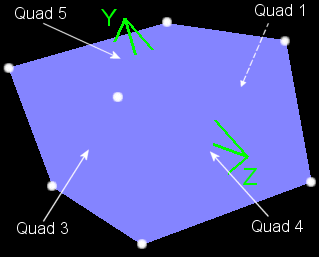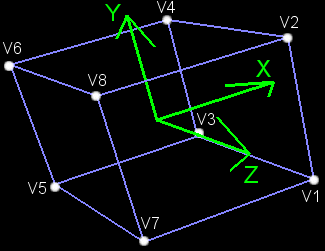Cube rendered with GL_FILL Cube rendered with a GL_LINE

You can remarks that I carry of the order of the glVertex calls. The order defines the face orientation, see Tutorial 5 : Face orientation for more information on this. One side of the face is front (GL_FRONT), the other is back (GL_BACK).

Remarks :
GL. in front of the OpenGl constant is for Jogl (don't need with Gl4java because GLAnimCanvas extends GLEnum).• ## Transformations

A transformations is either a translation, a rotation or a scale. The drawing is performed in the transform coordinate system.

We use a translation to move the pyramid to the left and the cube to the right. Without transformatrion, the cube and the pyramid will be superimposed.

• ### Translation

Before drawing the objects, we begin to translation the center of the coordinate system where the pyramid will be drawn.
To position the pyramid to the left, we just have to translate the axis system like this :
gl.glTranslatef(xTranslation, yTranslation, zTranslation)
For the pyramid, we translate from these values (-1.5f, 0.0f, -8.0f)
The coordinate system center will be translated to 1.5 units to the left and 8 units backwards. The raison why we translate along z axis is that initialy the coordinate system is on the near plane of the viewing volume. This is better explained in the Lesson 1.

To position the cube, there are differents solutions :

•  Method 1 : translate to the right, symmetrically to the pyramid
gl.glTranslatef(3.0f, 0.0f, 0.0f)
•  Method 2 : reset the view and move to the position of the cube
gl.glTranslatef(1.5f, 0.0f, zOffset)

glLoadIdentity reset transformations applyed to the coordinate system.

• ### Rotation

To rotate the system axis around its center, we use the command :
gl.glRotatef(angle, xAxis, yAxis, zAxis)
The *Axis parameters is a unit vector defining the axis of rotation (ĂŞ on the picture). It represents a unit vector. The angle parameter is the rotation angle in degree (θ on the picture).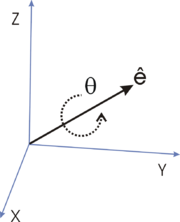Axis/Angle rotation, picture from Wikipedia

Here, we want the shape to rotate around the vertical axis.
Vertical axis is y, so the direction is (0, 1, 0), rotPyramid represents the rotation angle is degree :
gl.glRotatef(rotPyramid, 0.0f, 1.0f, 0.0f)    //rotation around y axis• ### Render

We have seen two differents render in the previous pictures ie face filled or render as line.

The rendering style is defined with :
gl.glPolygonMode(face, mode)
face parameter is either GL_FRONT_AND_BACK, GL_FRONT or GL_BACK
mode parameter is either GL_POINT, GL_LINE or GL_FILL

In this tutorial, shapes are render so :

•  GL_FRONT face seen we are in the outside of the shape.

•  GL_BACK  face seen we are in the  inside of the shape.

We will see in the Tutorial 5 : Face Orientation how OpenGl determine the front and the back face of an object.

• ### Hide a Face

We can hide a face (GL_FRONT or GL_BACK) to optimize drawing performance.
For this, enable culling mode represented as GL_CULL_FACE. To activate it, use the command glEnable. To face culled is specified with glCullFace.
gl.glCullFace(face)
gl.glEnable(GL_CULL_FACE)

Here is an exemple of a hidding the back face of the previous screen shots :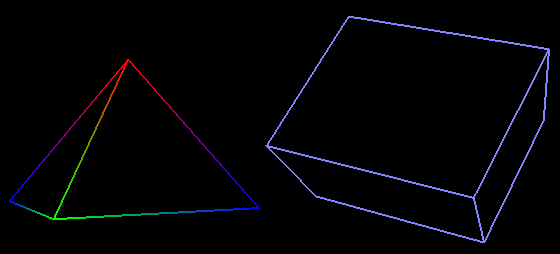Scene using glCullFace(GL_BACK)

This mode defines how the color is propagated around a vertex. To select a mode, we use :
mode is one of these two values :
GL_FLAT   : same color to all the face
GL_SMOOTH : gradation of colors around the vertex (colors is mixed between two vertices)

All the screen shots are taken with a smooth shading model. The following picture uses a flat rendering :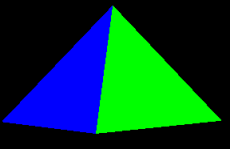• ### Line width

We can defines the width of the lines with :
gl.glLineWidth(width)

Width is a float that defines the width of the line. The default value is 1.f.

Exemple of a line width equal to 5.f :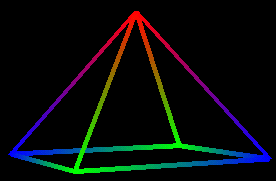Pyramid with a LineWidth equal to 5.f• ## Keys Summary

C : hide/view back face
R : switch between GL_LINE & GL_FILL render
P : pause the rotation of the shapes